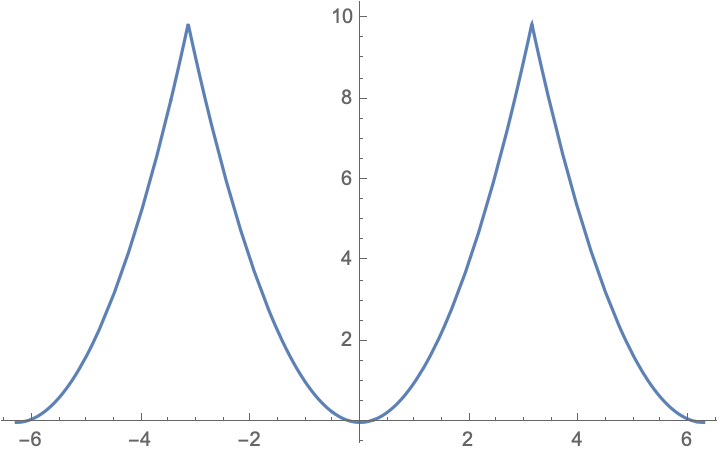# Why is $-\int_{-\infty}^\infty \log\left[1+2f'(x)(1-\cos\phi)\right]\,dx$ equal to $\phi^2$?

I came across this integral involving the derivative $$f'(x)$$ of the Fermi function $$f(x)=(1+e^x)^{-1}$$: $$I(\phi)=-\int_{-\infty}^\infty \log\left[1+2f'(x)(1-\cos\phi)\right]\,dx.$$ I'm pretty certain that $$I(\phi)=\phi^2$$ for $$|\phi|<\pi$$, periodically repeated, as in the plot. It means that only the $${\cal O}(\phi^2)$$ term in a Taylor expansion of the integrand has a nonzero contribution, but I am unable to prove this. (Mathematica returns a polylog function, and will not simplify it further.) Any help would be much appreciated, I'm hoping such a simple answer will have a simple derivation --- perhaps without needing special functions?• Why do you think it is $\phi^2$ ? Numerically, it does not seem so, I cannot reproduce your graph, and indeed the result is a combination of polylogs. Apr 17 '20 at 9:53
• apologies, typo corrected (minus sign); my colleague Omrie Ovdat just noticed that if you replace $\cos\phi$ by $\cos i\phi$, then Mathematica returns $-\phi^2$ --- so the inverted parabola without the periodic repetition. Apr 17 '20 at 10:18
• @user82588 -- presumably that is how Mathematica arrives at the answer; since the result is so simple, I was actually hoping there would be a more direct way of obtaining it, without the need for special functions, in particular because I hope to gain understanding for why only the $\phi^2$ survives integration --- in the physics problem where this integral appeared, the quadratic answer is meaningful and I would hope to understand its origin. Apr 17 '20 at 11:27

It is clear that $$I(0)=0$$, hence by Leibniz's integration rule, it suffices to show that $$\int_{-\infty}^\infty\frac{-2f'(x)\sin\phi}{1+2f'(x)(1-\cos\phi)}\,dx=2\phi,\qquad |\phi|<\pi.$$ We can assume, without loss of generality, that $$0<\phi<\pi$$. By a bit of algebra, we can rewrite the last equation as $$\int_{-\infty}^\infty\frac{e^x\sin\phi}{e^{2x}+2(\cos\phi)e^x+1}\,dx=\phi.$$ By the change of variables $$u=(e^x+\cos\phi)/\sin\phi$$, this becomes $$\int_{\frac{\cos\phi}{\sin\phi}}^\infty\frac{du}{u^2+1}=\phi,$$ which in turn can be verified by calculus: $$\int_{\frac{\cos\phi}{\sin\phi}}^\infty\frac{du}{u^2+1}=\arctan(\infty)-\arctan\left(\frac{\cos\phi}{\sin\phi}\right)=\frac{\pi}{2}-\left(\frac{\pi}{2}-\phi\right)=\phi.$$ The proof is complete.
We have $$I(t)=-\int_{-\infty}^\infty l_x(t)\,dx,$$ where $$l_x(t):=l(t):=\ln(1+2f'(x)(1-\cos t)).$$ Next, $$l_x''(t)=l''(t)=-\frac{2 e^x \left(c \left(e^{2 x}+1\right)+2 e^x\right)}{\left(2 c e^x+e^{2 x}+1\right)^2},$$ where $$c:=\cos t\in(-1,1]$$ for $$|t|<\pi$$. So, by substitution $$e^x=u$$, for $$c\in(-1,1]$$, $$I''(t)=-\int_{-\infty}^\infty l''_x(t)\,dx=2,$$ which implies $$I(t)=t^2$$ for $$|t|<\pi$$ (since $$l_x(0)=0=l'_x(0)$$ and hence $$I(0)=0=I'(0)$$).